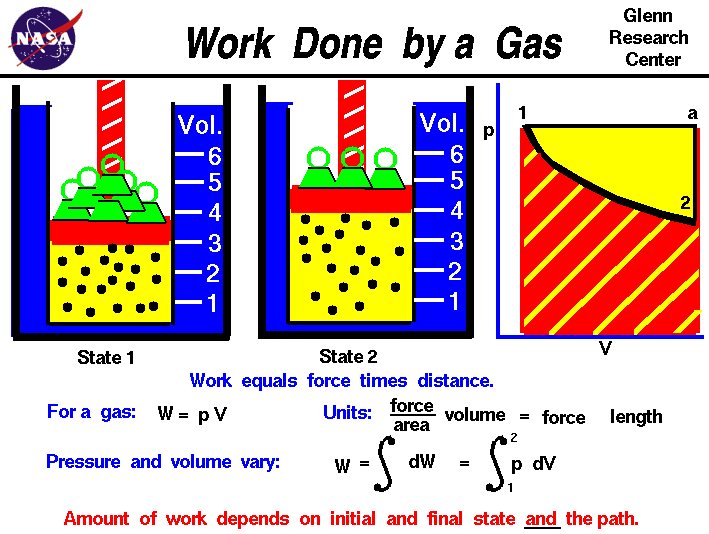• ×
الأربعاء 28 أكتوبر 2020 | 08-26-2020
×

# الشغل بواسطة الغاز Pressure-volume work# Work Done by a GasWhen a gas expands it does work on its surroundings. That work is equal to the area under the curve on a P-V diagram which describehs that expansion.Isobaric process:

Process at constant pressure:W = F D

W = ( F / A ) ( A D )

W = P ( AD )

W = P (V)

Work = area under the curve on a P-V diagram.

Work = area under the curve on a P-V diagram -- even if the process is not isobaric.Isometric processes:

Isochoric:

Constant volume:No work is done in an isometric process.

Isothermal process:

Process at constant temperature:We can keep the temperature constant by having the system in contact with a heat reservoir.Process with zero heat flow:

Insulated:The amount of work done will be less than for an isothermal process between the same two volumes. Notice that an adiabat is steeper than an isotherm.

Example:W = 2.5 atm-l

Now, what is an "atmosphere-liter"? It is a pressure (atm) multiplied by a volume (liter) so it must be some sort of work or energy. Now, this is just a units conversion question,

W = 2.5 atm-l [ 0.001 m3 / 1 l ] [ 1.013 x 105 Pa / 1 atm ]

W = 253 Pa-m3 [ (N/m2) / Pa ]

W = 253 N-m

W = 253 J

المصدر

1

2

## روابط التنزيل

من فضلك قم بكتابة تعليق لتظهر لك روابط التحميل

بواسطة :
08-15-2016 12:00 صباحاً  0  0  1.8K  21
التعليقات ( 0 )

0 | 66 | 5

0 | 107 | 4

0 | 97 | 7

4 | 140 | 25

7 | 143 | 16

2 | 146 | 81